0

# 汉诺塔 问题 python 解决

## 思考步骤

A—>B #借助B，帮助C拿到最后一个盘子
A—>C #最后一个盘子移动完成后，剩余的盘子已经全部到B上了
B—>C #将剩余的盘子移动到C上，完成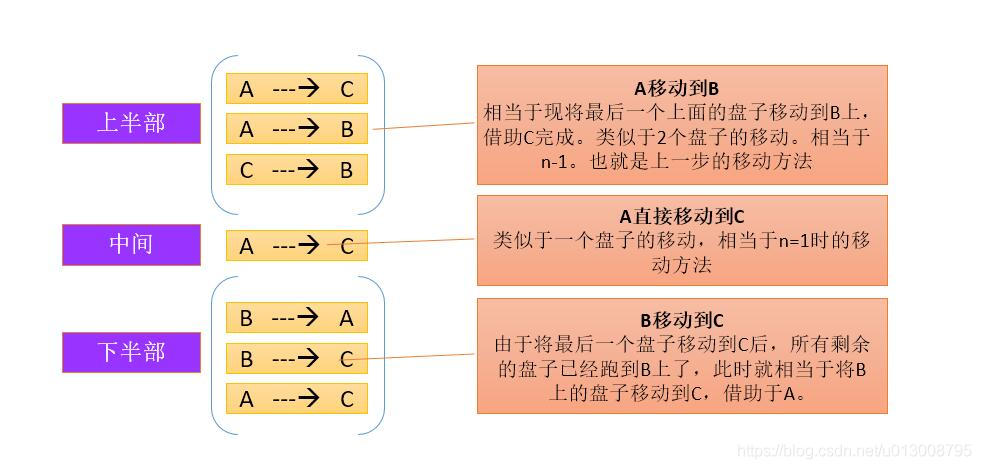(因为要将第n个大盘子移动的前提是 将 前n-1个小盘子 移动到另一个柱子上)

## 代码实现

``````defmove(n,a,b,c):if n==1:print(a,'--->',c)else:
move(n-1,a,c,b)print(a,"--->",c)
move(n-1,b,a,c)
move(3,"a","b","c")
``````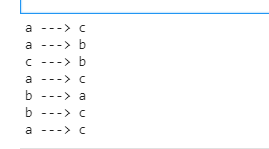``````defmove(n,a,b,c):if n==1:print(a,'--->',c)else:
move(n-1,a,c,b)print(a,"--->",c)
move(n-1,b,a,c)
move(64,"a","b","c")
``````

## 公式解决汉诺塔移动的次数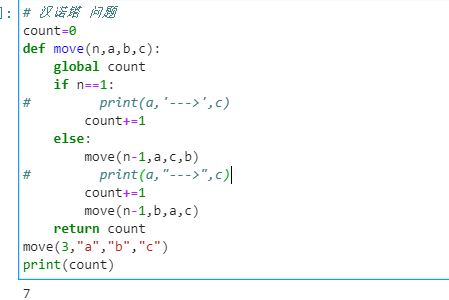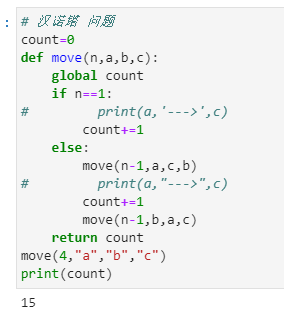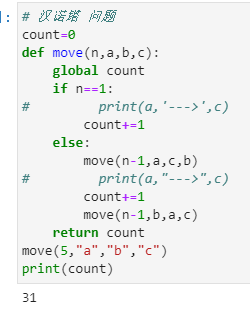XSWL 难道这不就是 通式 2^n-1 嘛

``````n=64print("汉诺塔移动步数:",2**n-1)
``````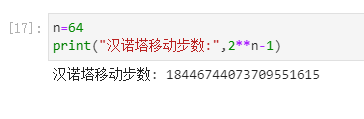### “汉诺塔 问题 python 解决”的评论:

##### 关于作者##### overfit同步小助手